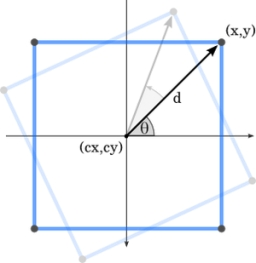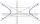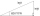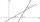# Coordinates of square vertices

I have coordinates of square vertices A / -3; 1/and B/1; 4 /. Find coordinates of vertices C and D, C 'and D'. Thanks Peter.

Result

x2 =  2.97
y2 =  -0.596
x3 =  -1.03
y3 =  -3.596
x4 =  -0.97
y4 =  8.596
x5 =  -4.97
y5 =  5.596

#### Solution:

$x_{ 0 } = -3 \ \\ y_{ 0 } = 1 \ \\ \ \\ x_{ 1 } = 1 \ \\ y_{ 1 } = 4 \ \\ \ \\ a = \sqrt{ (x_{ 0 }-x_{ 1 })^2+(y_{ 0 }-y_{ 1 })^2 } = \sqrt{ ((-3)-1)^2+(1-4)^2 } = 5 \ \\ \ \\ \tan α = \dfrac{ y_{ 0 }-y_{ 1 } }{ x_{ 0 }-y_{ 1 } } = \dfrac{ 1-4 }{ (-3)-4 } = \dfrac{ 3 }{ 7 } \doteq 0.4286 \ \\ \ \\ α = \arctan (\dfrac{ y_{ 0 }-y_{ 1 } }{ x_{ 0 }-y_{ 1 } } ) = \arctan (\dfrac{ 1-4 }{ (-3)-4 } ) \doteq 0.4049 \ rad \ \\ \ \\ dx = a \cdot \ \cos(α) = 5 \cdot \ \cos(0.4049) \doteq 4.5957 \ \\ dy = a \cdot \ \sin(α) = 5 \cdot \ \sin(0.4049) \doteq 1.9696 \ \\ \ \\ x_{ 2 } = x_{ 1 } + dy = 1 + 1.9696 \doteq 2.9696 = 2.97$
$y_{2} =y_{ 2 } = y_{ 1 } - dx = 4 - 4.5957 \doteq -0.5957 = -0.596$
$x_{ 3 } = x_{ 0 } + dy = (-3) + 1.9696 \doteq -1.0304 = -1.03$
$y_{ 3 } = y_{ 0 } - dx = 1 - 4.5957 \doteq -3.5957 = -3.596$
$x_{4} =x_{ 4 } = x_{ 1 } - dy = 1 - 1.9696 \doteq -0.9696 = -0.97$
$y_{4} =y_{ 4 } = y_{ 1 } + dx = 4 + 4.5957 \doteq 8.5957 = 8.596$
$x_{ 5 } = x_{ 0 } - dy = (-3) - 1.9696 \doteq -4.9696 = -4.97$
$y_{ 5 } = y_{ 0 } + dx = 1 + 4.5957 \doteq 5.5957= 5.596 \ \\ \ \\ a_{ 2 } = \sqrt{ (x_{ 0 }-x_{ 3 })^2+(y_{ 0 }-y_{ 3 })^2 } = \sqrt{ ((-3)-(-1.0304))^2+(1-(-3.5957))^2 } \doteq 5.0004 \ \\ a_{ 3 } = \sqrt{ (x_{ 1 }-x_{ 2 })^2+(y_{ 1 }-y_{ 2 })^2 } = \sqrt{ (1-2.9696)^2+(4-(-0.5957))^2 } \doteq 5.0004 \ \\ a_{ 4 } = \sqrt{ (x_{ 2 }-x_{ 3 })^2+(y_{ 3 }-y_{ 2 })^2 } = \sqrt{ (2.9696-(-1.0304))^2+((-3.5957)-(-0.5957))^2 } = 5 \ \\ \ \\ b_{ 2 } = \sqrt{ (x_{ 0 }-x_{ 5 })^2+(y_{ 0 }-y_{ 5 })^2 } = \sqrt{ ((-3)-(-4.9696))^2+(1-5.5957)^2 } \doteq 5.0002 \ \\ b_{ 3 } = \sqrt{ (x_{ 1 }-x_{ 4 })^2+(y_{ 1 }-y_{ 4 })^2 } = \sqrt{ (1-(-0.9696))^2+(4-8.5957)^2 } \doteq 5.0004 \ \\ b_{ 4 } = \sqrt{ (x_{ 4 }-x_{ 5 })^2+(y_{ 4 }-y_{ 5 })^2 } = \sqrt{ ((-0.9696)-(-4.9696))^2+(8.5957-5.5957)^2 } \doteq 5.0002$Our examples were largely sent or created by pupils and students themselves. Therefore, we would be pleased if you could send us any errors you found, spelling mistakes, or rephasing the example. Thank you!

Leave us a comment of this math problem and its solution (i.e. if it is still somewhat unclear...):Be the first to comment!#### Following knowledge from mathematics are needed to solve this word math problem:

For Basic calculations in analytic geometry is helpful line slope calculator. From coordinates of two points in the plane it calculate slope, normal and parametric line equation(s), slope, directional angle, direction vector, the length of segment, intersections the coordinate axes etc. Pythagorean theorem is the base for the right triangle calculator. Most natural application of trigonometry and trigonometric functions is a calculation of the triangles. Common and less common calculations of different types of triangles offers our triangle calculator. Word trigonometry comes from Greek and literally means triangle calculation.

## Next similar math problems:

1. Triangle IRTIn isosceles right triangle ABC with right angle at vertex C is coordinates: A (-1, 2); C (-5, -2) Calculate the length of segment AB.
2. Unit vector 2DDetermine coordinates of unit vector to vector AB if A[-6; 8], B[-18; 10].Given that P = (5, 8) and Q = (6, 9), find the component form and magnitude of vector PQ.
4. HyperbolaFind the equation of hyperbola that passes through the point M [30; 24] and has focal points at F1 [0; 4 sqrt 6], F2 [0; -4 sqrt 6].
5. Vector 7Given vector OA(12,16) and vector OB(4,1). Find vector AB and vector |A|.
6. Euclid2In right triangle ABC with right angle at C is given side a=27 and height v=12. Calculate the perimeter of the triangle.
7. ABS CNCalculate the absolute value of complex number -15-29i.
8. The fenceI'm building a fence. Late is rounded up in semicircle. The tops of late in the field between the columns are to copy an imaginary circle. The tip of the first and last lath in the field is a circle whose radius is unknown. The length of the circle chord i
9. RightDetermine angles of the right triangle with the hypotenuse c and legs a, b, if: ?
10. The ditchDitch with cross section of an isosceles trapezoid with bases 2m 6m are deep 1.5m. How long is the slope of the ditch?
11. DistanceWha is the distance between the origin and the point (18; 22)?
12. CardsSuppose that are three cards in the hats. One is red on both sides, one of which is black on both sides, and a third one side red and the second black. We are pulled out of a hat randomly one card and we see that one side of it is red. What is the probabi
13. One sideOne side is 36 long with a 15° incline. What is the height at the end of that side?
14. Theorem proveWe want to prove the sentence: If the natural number n is divisible by six, then n is divisible by three. From what assumption we started?
15. Angle between linesCalculate the angle between these two lines: ? ?
16. TrigonometryIs true equality? ?
17. CotangentIf the angle α is acute, and cotg α = 1/3. Determine the value of sin α, cos α, tg α.